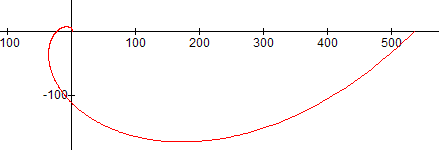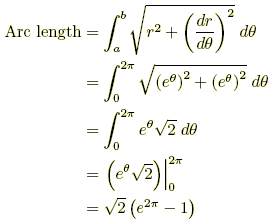index: click on a letter A B C D E F G H I J K L M N O P Q R S T U V W X Y Z A to Z index index: subject areas numbers & symbols sets, logic, proofs geometry algebra trigonometry advanced algebra & pre-calculus calculus advanced topics probability & statistics real world applications multimedia entrieswww.mathwords.com about mathwords website feedback

Arc Length of a Curve

The length of a curve or line.

The length of an arc can be found by one of the formulas below for any differentiable curve defined by rectangular, polar, or parametric equations.

For the length of a circular arc, see arc of a circle.

 Formula: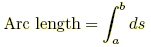where a and b represent x, y, t, or θ-values as appropriate, and ds can be found as follows. 1. In rectangular form, use whichever of the following is easier: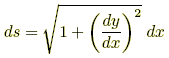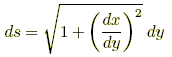2. In parametric form, use3. In polar form, use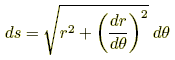Example 1: Rectangular Find the length of an arc of the curve y = (1/6) x3 + (1/2) x–1 from x = 1 to x = 2.Example 2: Parametric Find the length of the arc in one period of the cycloid x = t – sin t, y = 1 – cos t. The values of t run from 0 to 2π.Example 3: Polar Find the length of the first rotation of the logarithmic spiral r = eθ. The values of θ run from 0 to 2π.